﻿ Rotating mixer with SRF function - XSim

# Rotating mixer with SRF function

OpenFOAM 4.x

## Case directory

\$FOAM_TUTORIALS/incompressible/SRFSimpleFoam/mixer

## Summary

A mixer rotating at 1000 rpm in a cylinder around the Z-axis is calculated. The rotation of the computational domain is modeled by adding centrifugal and Coriolis forces (SRF function).

Fluid flows in from the region "inlet" (Z-maximum surface) at (0, 0, -10) m/s and out from the region "outlet" (Z-minimum surface). The region "outerWall" is set to a stationary wall, and the mixer surface (region "innerWall") is set to a no-slip wall. Only a quarter of the geometry is modeled, and cyclic boundaries are used on the dividing surfaces ("cyclic_half0" and "cyclic_half1") to calculate the entire flow.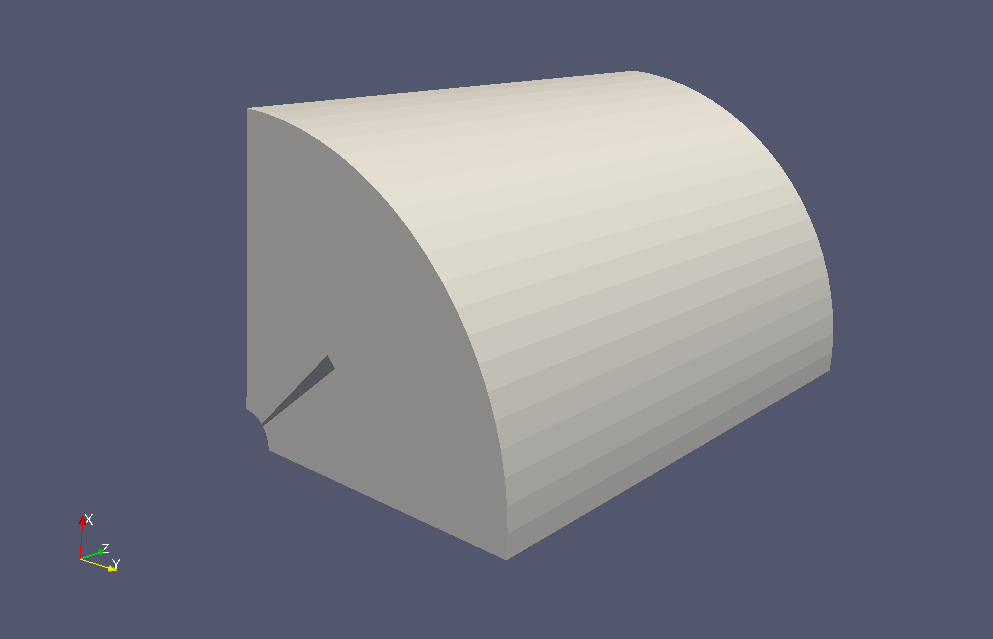Model geometryModel geometry (Flow cross-sectional direction)

The rotation axis and the rotation speed are specified in the file constant/SRFProperties as shown below.

```SRFModel        rpm;
origin          (0 0 0);
axis            (0 0 1);
rpmCoeffs
{
rpm         1000;
}
```

The boundary condition of the flow velocity for the rotating coordinate system is specified in the file 0/Urel. However, for the region "inlet" and the region "outerWall", the SRFVelocity type is set as shown below, and the velocity for the stationary coordinate system is set as "relative no;".

```inlet
{
type            SRFVelocity;
inletValue      uniform (0 0 -10);
relative        no;
value           uniform (0 0 0);
}

outerWall
{
type            SRFVelocity;
inletValue      uniform (0 0 0);
relative        no;
value           uniform (0 0 0);
}
```

The k-ω SST model is used as the turbulence model.

The meshes are as follows, and the number of mesh is 33600.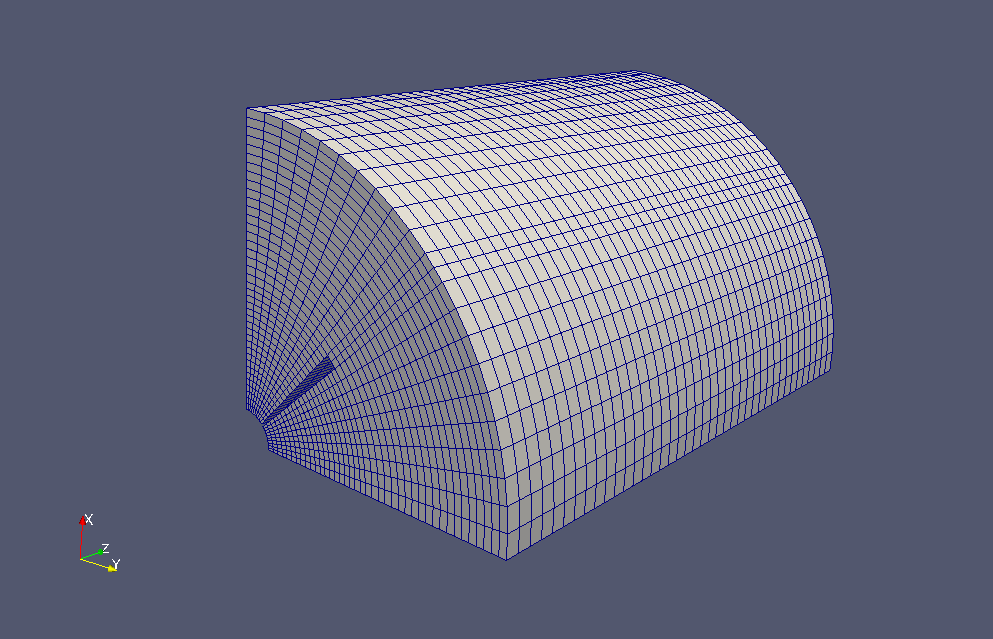Meshes

If you want to visualize the flow velocity in the inertia system, check "U" in the "Properties" tab on ParaView. Note that the data at time 0 does not have "U"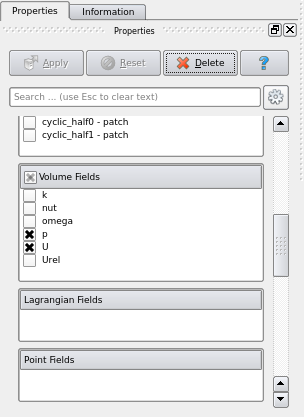Check "U"

The calculation result is as follows.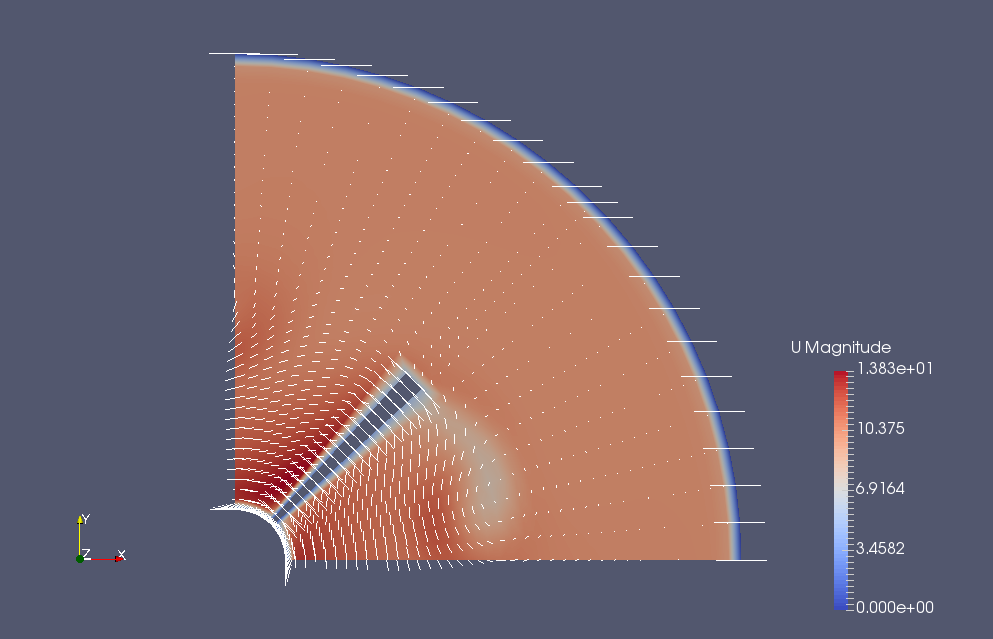Flow velocity in the cross-sectional direction near the center of the cylinder (U)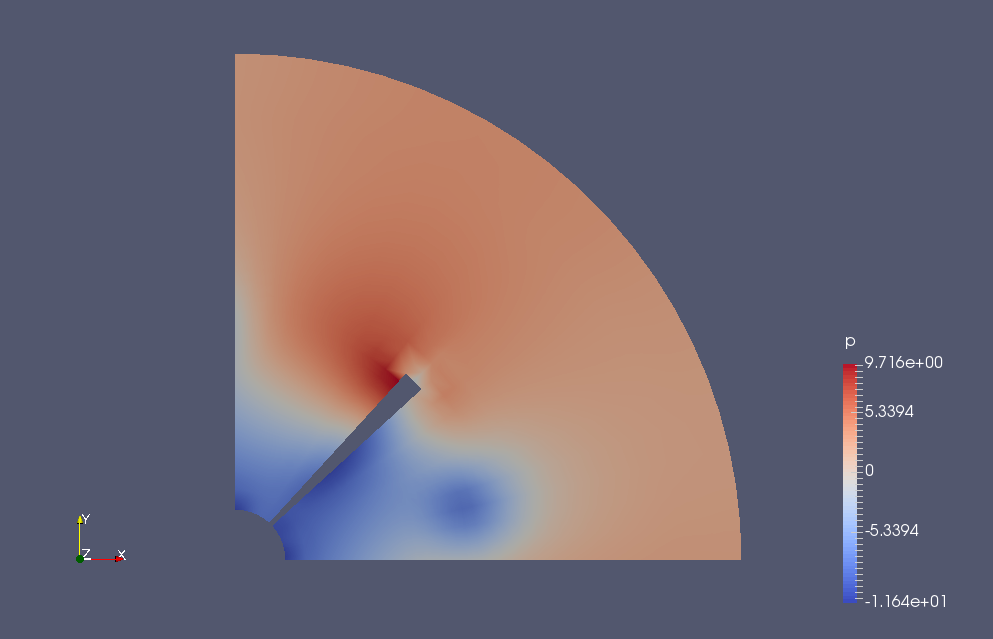Pressure in the cross-sectional direction near the center of the cylinder (p)

## Commands

cp -r \$FOAM_TUTORIALS/incompressible/SRFSimpleFoam/mixer mixer
cd mixer

blockMesh
SRFSimpleFoam

paraFoam

## Calculation time

2 minutes 12.34 seconds *Single, Inter(R) Core(TM) i7-2600 CPU @ 3.40GHz 3.40GHz

## Reference

OpenFOAM －回転領域を含む流体計算 (Rotating Geometry)－, Fumiya Nozaki